# IIT JAM Biotechnology Mock Test 1

## 60 Questions MCQ Test Mock Test Series of IIT JAM Biotechnology | IIT JAM Biotechnology Mock Test 1

Description
Attempt IIT JAM Biotechnology Mock Test 1 | 60 questions in 180 minutes | Mock test for IIT JAM preparation | Free important questions MCQ to study Mock Test Series of IIT JAM Biotechnology for IIT JAM Exam | Download free PDF with solutions
QUESTION: 1

### Identify (1R, 2R)-1, 2-diphenyethane-1, 2-diol.

Solution: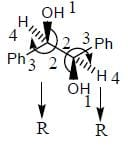QUESTION: 2

### The order of decreasing acidity of the following compound is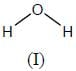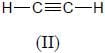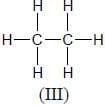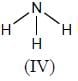Solution:

Conjugate base stability order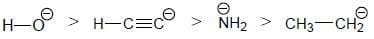Acidity increases with increase in conjugagte base statbility

QUESTION: 3

### Consider the arrangement as shown in figure. Spring constants are k1 and k2. All surfaces are frictionless. If block is slightly displaced, what is the angular frequency of oscillation?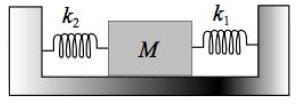Solution:

This arrangement is parallel arrangement of springs.
So, equivalent spring constant (Keq) = k1 + k2
Angular frequency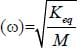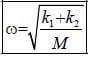QUESTION: 4

What should be the angle 'a', so that a light ray retraces its path after third reflection (θ = 300)?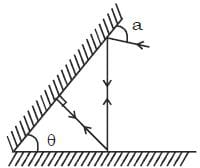Solution:

Following laws of reflection, if the ray retraces its path :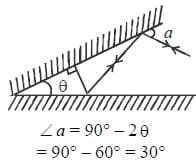QUESTION: 5

The theory of cell lineage ‘omnis cellula e cellula’ was proposed by,

Solution:

Rudolf Virchow, in the year 1855, proposed an extension to the cell theory (theory of cell lineage), omnis cellula e cellula (means, all living cells arise from pre-existing cells).

QUESTION: 6

All of the under-mentioned factors affect membrane fluidity except,

Solution:

Temperature: Lipid exists in a relatively fluid state at 37°C and at lower temperature, it converts to frozen crystal gel.
Fatty acid chain length: The shorter the fatty acid chain length, the lower is the melting temperature of the phospholipid bilayer.
Saturation: The greater the degree of unsaturation of the fatty acid, the lower is the melting temperature of the lipid bilayer.
pH: There is no such effect of pH on membrane permeability.

QUESTION: 7

Shell coiling in Limnaea shows maternal effect in the progenies. Right-handed shell coiling is controlled by D and is dominant to left-handed coiling which is control by d. A cross between two Limnaea produces progenies of all left-handed. Which of the following is TRUE for the above cross?

Solution:

Genotype of the mother determine the progeny phenotype.

QUESTION: 8

Inbred lines of two mutant plants, both bearing white flowers, were crossed. The F1 plants were all red coloured flowers. Selfing the F1 plants, produced progeny with phenotypes of red and white coloured flowers in 9: 7 ratio. Based on this information, which one of the following conclusions is correct?

Solution:

A dihybrid cross with two gene loci. Mutations are complement to one another

QUESTION: 9

Histamine is one of the most potent mediators of inflammation. It acts by

Solution:

Histamine is a vasodilator so it causes vasodilation & also increases tissue permeability.

QUESTION: 10

Which among the following will NOT participate in innate immunity?

Solution:

Mediators of innate immunity include.
· Physiological barriers such as gastric HCl.
· Chemical barriers such as complement system, interferons, collectins etc.
· While abs of any class are mediators of adaptive immunity,  so IgM will mediate adaptive immunity.

QUESTION: 11

The product R in the following reaction is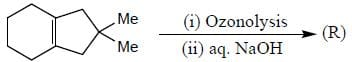Solution: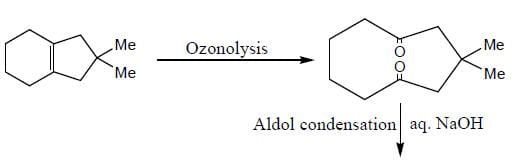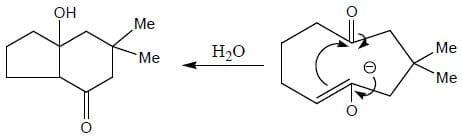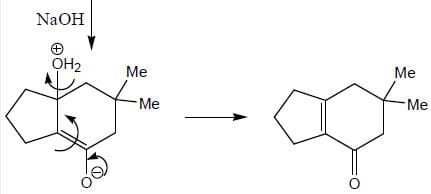QUESTION: 12

Match list - I with list - II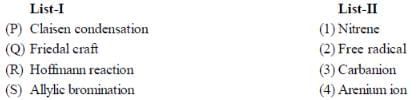Solution:

Claisen condensation occur through carbanion.
Arenium ion form in Friedal craft reaction.
Nitrene intermediate formed in Hoffmann reaction.
Allylic radical is more stable. So, Allylic bromination occur.

QUESTION: 13

Following statements are true regarding primary immune response except,

Solution:

Features of primary immune response are -
· It begins on 1st exposure to an Ag.
· It is applicable only to adaptive immunity & NOT to innate immunity.
· It does not use immune memory.
· It is applicable to both humoral & cell mediated immunity.
So, statement (b) is not true.

QUESTION: 14

RAG recombinase is responsible for the formation of specific immune receptors. This process occurs in

Solution:

The recombination-activating genes (RAGs) encode enzymes that play important roles in the rearrangement and recombination of the genes encoding immunoglobulin and T cell receptor molecules. Their cellular expression is restricted to lymphocytes during their developmental stage.

QUESTION: 15

Consider the combination of lenses as given below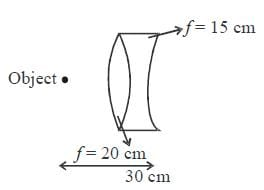Focal length of convex and concave lenses are 20 cm and 15 cm respectively. Distance from the combination and nature of image of an object situated at 30 cm from the lens combination is

Solution: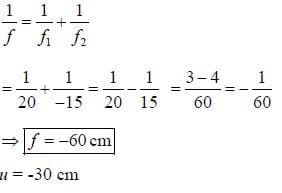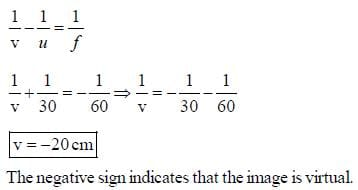QUESTION: 16

A convexo – concave lens with radius of curvature of convex surface 15 cm and of concave surface 30 cm is  made of material of refractive index 1.5. The nature and focal length of the lens is {convex surface is in front of the object}

Solution: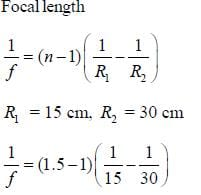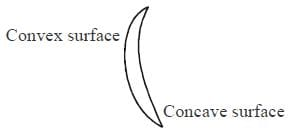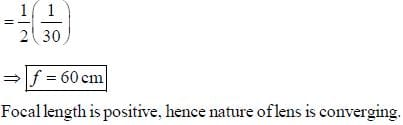QUESTION: 17

Consider two simple harmonic motions represented by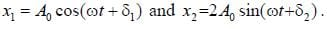At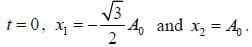If these two simple harmonics are combined, what is the resultant amplitude:

Solution: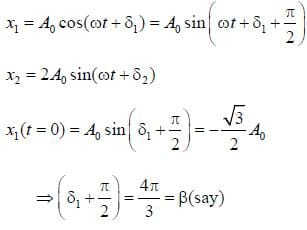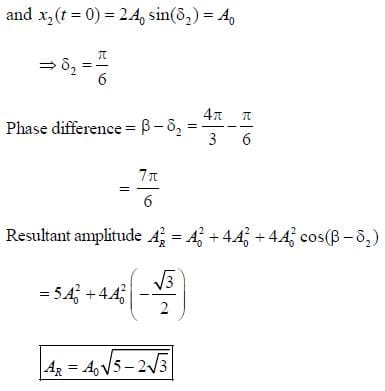QUESTION: 18

A kingfisher is flying 36 m high from the water surface of a pond. A fish is 36 m deep in water from the water surface. At what depth from the water surface, the fish seems to be to the kingfisher {refractive index of water is 4/3}

Solution: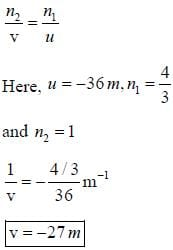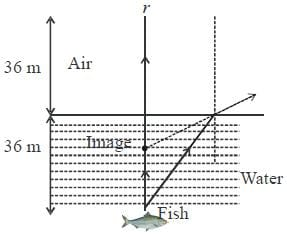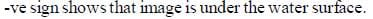QUESTION: 19

Microtubule polymerization can be inhibited by:

Solution:

Microtubules continuously undergo a process of dynamic instability, whereby microtubule polymerization on the plus end competes with depolymerization at the minus end. The polymerization of microtubule can be inhibited by colchicine, which is an alkaloid.

QUESTION: 20

The most abundant phospholipid in plasma membrane is,

Solution:

Glycerophospholipids are the most abundant phospholipids found in the plasma membrane amongst which, phophatidylcholine is the most abundant in plasma membrane.

QUESTION: 21

Choose the correct order of the molecules based on their permeability across the plasma membrane in increasing order.

Solution:

Ions (here, K+) cannot freely cross plasma membrane. The plasma membrane is almost impermeable or say least permeable to large, uncharged polar molecules (here, sucrose). Small, uncharged polar molecules (here, glycerol) show permeability across the plasma membrane. Small, non polar molecules (here, N2) are highly permeable across the plasma membrane.

QUESTION: 22

Nuclear-localization signals are rich in:

Solution:

Nuclear-localization signals direct the nuclear proteins to the nucleus and acts as a binding site for importin. These signals are Lys rich.

QUESTION: 23

If a heterozygous blood type A parent and a heterozygous blood type B parent mate, one-quarter of their offsprings will have blood type AB. This is an example of which type of inheritance?

Solution:

The blood type is specified by three type of alleles IA, IB and i, where i is recessive to both IA and IB. IA and IB are co-dominant alleles which means that both of them get expressed in the offspring.

QUESTION: 24

Two individuals heterozygous for a recessive lethal allele are expressed in uteri. What genotypic ratio (homozygous dominant : heterozygous : homozygous recessive) would you expect to observe in the off spring?

Solution:

The trait is lethal when both recessive alleles are expressed.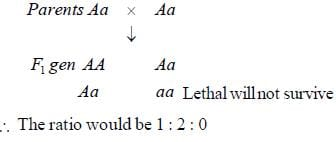QUESTION: 25

If the allele encoding polydactyly is dominant, why do most people have five fingers?

Solution:

Whether an allele is dominant or recessive does not correspond to its pre-dominance in the population. This means a dominant allele can be very rare in a population and not many people express it.

QUESTION: 26

How many offspring genotypes are expected in a trihybrid cross between parents heterozygous for all three traits. The traits behave in dominant and recessive pattern. Also give the number of phenotypes.

Solution:

The number of genotypes in trihybrid cross is given by 3where n = number of genes
Number of genes here = 3
∴ 3n = 33 =  27
Hence 27 genotypes.

QUESTION: 27

Type-II hypersensitivity reaction is mainly mediated by

Solution:

Type-II hypersensitivity reaction is an antibody mediated process in which IgG and IgM antibodies are directed against antigens on cells (such as circulating red blood cells) or extracellular material (such as basement membrane).

QUESTION: 28

The most dominant antibody in body secretions was run on SDS-PAGE. The total number of bands obtained will be

Solution:

IgA is the most dominant secreted antibody in the body. It contains H-chain, L-chain, J-chain and a secretory component. Thus, on SDS-PAGE one will see 4 bands.

QUESTION: 29

An Ab molecule such as IgG can be digested using certain proteases. Chose the correct statement about the process

Solution: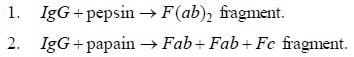QUESTION: 30

Which among the following will not occur during B-cell development?

Solution:

Somatic hypermutation & affinity maturation occur after B-cell activation.

*Multiple options can be correct
QUESTION: 31

Select the correct option regarding given properties

Solution:

In option (d), correct order of acidity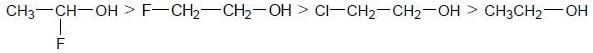*Multiple options can be correct
QUESTION: 32

Select the correct option regarding their products

Solution: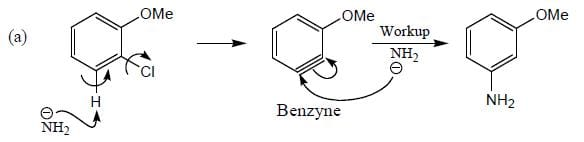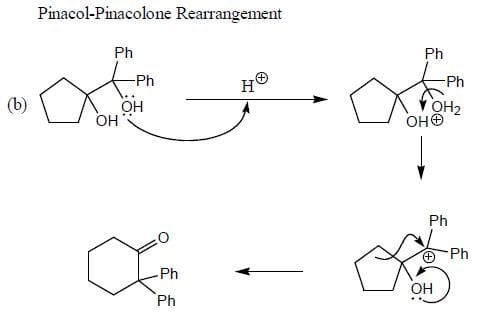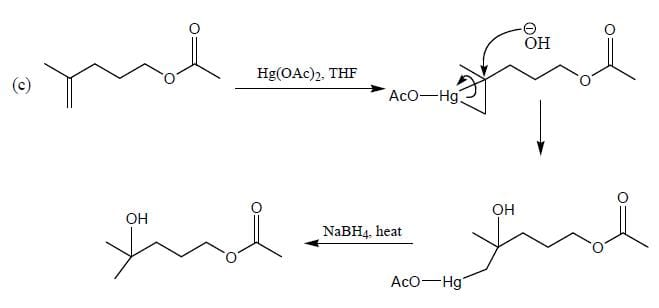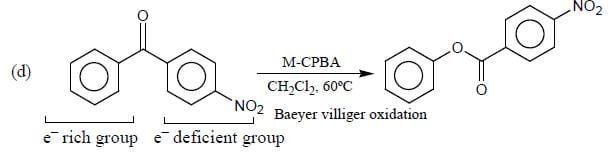*Multiple options can be correct
QUESTION: 33

Velocity of sound waves is

Solution:

Sound waves need medium to propagate. In gases, molecules are farther apart and that’s why it is hard for sound waves to travel in gases. Same reasoning for liquids and solids.

*Multiple options can be correct
QUESTION: 34

If Young’s double slit experiment is performed with white light then

Solution:

White light is collection of light of different wavelengts. At centre no path difference will happen hence white fringe. Also no dark fringes will occur. Also minimum wavelength light i.e., violet will be nearest to central fringe.

*Multiple options can be correct
QUESTION: 35

RBCs are used as the model for plasma membrane related studies because,

Solution:

The plasma membrane of human erythrocyte (red blood cell) is the most studied of all and is generally used as the model for plasma membrane related studies. The reasons underlying this choice include,
* RBCs are inexpensive and readily available.
* They are present as single cells, thus, reducing the effort of dissociating it from complex tissues.
* RBCs are simple, as they lack nuclear and cytoplasmic membranes. (Nuclear and cytoplasmic membranes are known to contaminate plasma membrane preparations from other cells.

*Multiple options can be correct
QUESTION: 36

The major phospholipids that are present in plasma membrane include,

Solution:

The plasma membrane of animal cells contains four major phospholipids,
1.    Phophatidylcholine
2.    Phophatidylserine
3.    Phophatidylethanolamine
4.    Sphingomyelin
Cerebroside and Ganglioside are examples of glycolipids and are found exclusively in the outer leaflet of the plasma membrane

*Multiple options can be correct
QUESTION: 37

Solution:

The Philadelphia chromosome is a truncated chromosome 22 which is a result of translocation between chromosome 9 and chromosome 22. It causes chronic myeloid leukemia.

*Multiple options can be correct
QUESTION: 38

Which of the following is true for a Barr body?

Solution:

Barr body is the inactive X chromosome in a female somatic cell. This process is called lyonisation after the Lyon hypothesis which states that cell with multiple X chromosomes have all but one X-chromosome inactivated. This happens during mammalian embryogenesis.

*Multiple options can be correct
QUESTION: 39

Choose the correct example that represent autoimmunity as well as hypersensitivity.

Solution:

Rheumatoid arthritis and systemic lupus erythematosus (SLE) are diseases that are autoimmune in nature but also lead to type - III hypersensitivity. While Hashimoto’s thyroiditis is only an autoimmune disease and  contact dermatitis is example of  only type-IV hypersensitivity.

*Multiple options can be correct
QUESTION: 40

Find correct statements about MHC genes

Solution:

Features of MHC genes include.
·    They are polygenic as well as polymorphic.
·    They are involved in identification of cells & tissues as self & non-self. Thus, called as histocompatibility complex.

*Answer can only contain numeric values
QUESTION: 41

Number of 1H NMR signals in the following compound (X) ______________ (Answer in integer)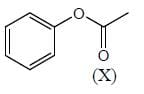Solution: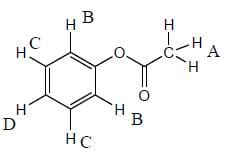*Answer can only contain numeric values
QUESTION: 42

If the frequency of air column in 2nd overtone mode in a closed organ pipe of length l1 is equal to the 2nd harmonic mode of the open organ pipe of length l2, then ratio of lengths l1/l2 is(upto tow decimal places) _____________(Round off to two decimal places)

Solution:

Modes of vibration for closed organ pipe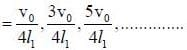Modes of vibration in open organ pipe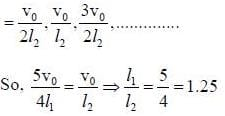*Answer can only contain numeric values
QUESTION: 43

If the work done by a human heart and an eyelid is 0.5J per beat and 0.025J per blink with frequency of 1.25 Hz and 2 Hz, respectively. The heart can work fine for ___________minutes at the cost of closing eyes for 4 hours. (give the answer in minutes) [Answer upto 2 decimal places]

Solution:

Let us say that the  heart can work 't' hours longer
⇒ work done by eyelids in 4 hrs = work done by heart in 't' hours
Remember, humans have two eyelids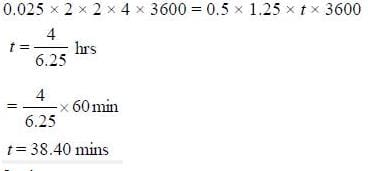*Answer can only contain numeric values
QUESTION: 44

A sound source of frequency 760 Hz and an observer are moving in the same direction with velocities 40 m/s and 20 m/s, respectively. The frequency of sound heard by the observer is ______Hz. (velocity of sound in air is 340 m/s). [Nearest integer]

Solution:

Actual frequency of source (v) = 760 Hz.
Velocity of source (vs) = 40m/s
Velocity of observer (v0) = 20 m/s
Velocity of sound (v) = 340 m/s
Frequency  heard by observer (v') is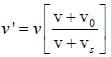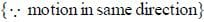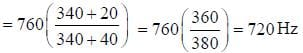*Answer can only contain numeric values
QUESTION: 45

In a double slit experiment, the separation between the slits is 1.00 mm, the wavelength of light used is 6000Å and the distance of the screen from the slits is 1.0 m. The number of bright fringes formed in 1.2 cm width on the screen is ____. [Answer is an integer]

Solution: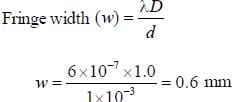Number of fringes in 1.2 cm width =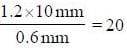Half of these will be dark fringes and half bright fringes
Hence number of bright fringes is = 20

*Answer can only contain numeric values
QUESTION: 46

A cell in G1 of Interphase has 12 chromosomes. In Anaphase-I of meiosis, the number of DNA molecules per cell will be ___________

Solution:

If a cell in G1 of Interphase has 12 chromosomes means it has 12 DNA molecules. In Anaphase-I of meiosis, this cell will contain 12× 2 = 24 DNA molecules.

*Answer can only contain numeric values
QUESTION: 47

The sum of ratio of CD4 : CD8 T - cells in human blood is ___

Solution:

Ratio of CD4 : CD8 T-cells in human blood is approximately 2 : 1.

*Answer can only contain numeric values
QUESTION: 48

200 individuals in a population carry the mutant alleles. However, only 160 individuals show the mutant phenotype and the remaining 40 individuals are normal despite the presence of mutant allele. The penetrance of the mutant allele in the population  will be _______________%

Solution:

Penetrance is the percent of people showing the phenotypes.

*Answer can only contain numeric values
QUESTION: 49

In a cross AABBCC × aabbcc, the number of different kinds of F2 genotypes will be _____

Solution: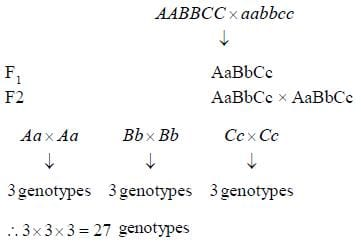*Answer can only contain numeric values
QUESTION: 50

The number of gametes  produced by individuals of PPQqRr genotype will be _______

Solution:

PQR, PqR, PQr, Pqr.

*Answer can only contain numeric values
QUESTION: 51

The number of nucleosomes present in a 30 nm solenoid structure of a chromatin are ____ (Answer in integer)

Solution:

According to solenoid structure of a chromatin, the 10 nm fibre of nucleosomes gets coiled upon itself to form a 30 nm wide helix. This 30 nm structure is called as solenoid. It has 5 or 6 nucleosomes per helix. The histone N-terminal tails direct the DNA to wrap around the histone octamer disc.

*Answer can only contain numeric values
QUESTION: 52

A spring block system of spring constant ' k ' and mass 'M' has harmonic angular frequency ω1. If the spring is now cut in half and the two pieces are now connected as shown in figure, angular frequency becomes ω2. The ratio of the two frequencies ω12 is ___. (Upto two decimal places)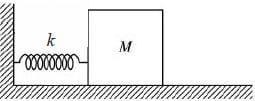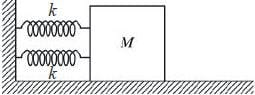Solution: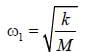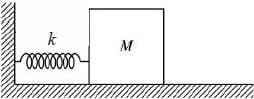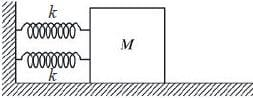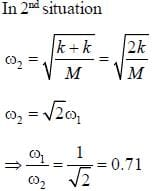*Answer can only contain numeric values
QUESTION: 53

A light source is fixed at the bottom of a 10 m deep lake. Minimum area of a canvas by which if we cover the lake no light will come outside the lake is ___ (in m2) [Answer upto two decimal places] (take refractive index of lake water to be √2). [Hint: Assume the centre of the canvas to be above the light source]

Solution: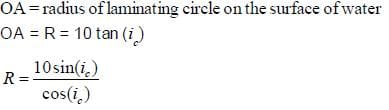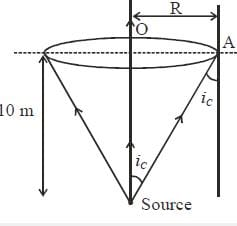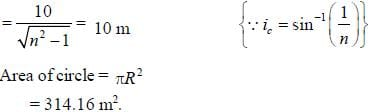*Answer can only contain numeric values
QUESTION: 54

A convex lens of focal length 30 cm and a concave lens of focal length 10 cm are kept at a distance d apart in the air. If light rays parallel to the principal axis are falling on the convex lens, the value of d for which light rays going out from the concave lens are also parallel to the principal axis  is ______ (give your answer in cms) [the answer is an integer]

Solution:

Parallel rays coming on the convex lens will converge on the focus, i.e.. 30 cm right to the convexlens. If these rays seem to be approaching the focus of concave lens, they will emerge as parallel rays.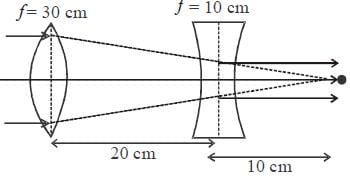So. the distance between lenses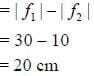*Answer can only contain numeric values
QUESTION: 55

When light rays pass through a prism of prism angle 60°, it suffers a maximum deviation of 30°. The refractive index of the material of prism is ____. (upto two decimals).

Solution: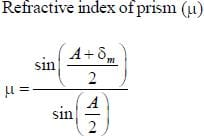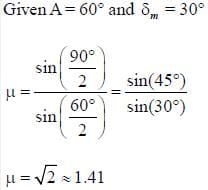*Answer can only contain numeric values
QUESTION: 56

A DNA segment has 120 adenine and 120 cytosine bases. The total number of nucleotides present in the segment are ________________ (Answer in integer)

Solution:

According to Chargaff rule.
Adenine (A) is paired to Thymine (T)
Cytosine (C) is paired to Guanine (G)
Here,
Cytosine residues = 120
[A] = [T]
[A= 120] = [T = 120]
[C] = [G]
[C = 120] = [G =120]
Therefore,
Total number of nucleotides = [A] + [T] + [C] + [G]
= 120 x 4 = 480

*Answer can only contain numeric values
QUESTION: 57

IgM  was put to SDS-PAGE, the respective number of bands will be  _________

Solution:

IgM has 3 different types of polypeptide in its structure i.e., H-chain, L-chain & J-chain. So, it will show 3 bands.

*Answer can only contain numeric values
QUESTION: 58

Two true-breeding lizards were crossed. But 2 mutant traits were found in the parents short tail and bent claws. The F1, lizards were all wild type in appearance. The F1, females were test crossed and the offspring obtained were :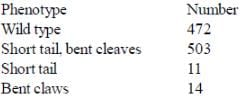The map distance between the two genes will be __________ cM

Solution: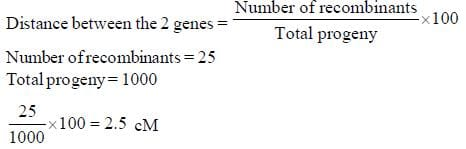*Answer can only contain numeric values
QUESTION: 59

In a population of plants, the shortest plants were 6 cm and the tallest were 24 cm. Three gene pairs controlled plant height. If homozygous 18 cm and 24 cm plants are crossed, the height of the F1 progeny  would be ____________cM. Assume that there is no environmental influence on the trait.

Solution:

The shortest and tallest plants are two extreme phenotypes :
aabbcc = 6 cm
AABBCC = 24 cm
Base height, when no additive allele is present = 6 cm
∴ Height contributed by each additive allele ⇒ 24 - 6 = 18 cm
⇒ 18 / 6 = 3 cm
A = B = C = 3 cm
The number of additive alleles in plant of height 18 cm contain = 4.
Since it is homozygous, it can be either AABBcc, AAbbCC or aaBBCC,
Thus, AABBcc x AABBCC → AABBCc.
The height of this plant will be 15 + 6 = 21 cm.

*Answer can only contain numeric values
QUESTION: 60

A complementation chart is given below. The number of genes defined by these mutations are ____ ((+) indicates complementation, (-) indicates failure to complement).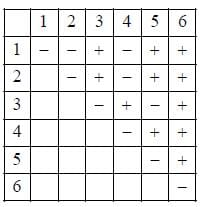Solution:

1, 2 and 4 are in one gene, 3 and 5 are in one gene, 6 is in a separate gene.
∴ Three genes are defined by these mutations.Use Code STAYHOME200 and get INR 200 additional OFF Use Coupon Code